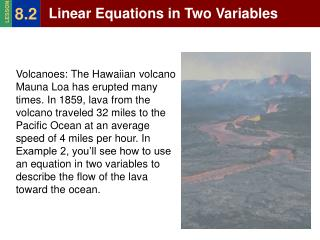DownloadDownload PresentationLinear Equations in Two Variables

Linear Equations in Two Variables

Download PresentationLinear Equations in Two Variables

- - - - - - - - - - - - - - - - - - - - - - - - - - - E N D - - - - - - - - - - - - - - - - - - - - - - - - - - -
Presentation Transcript

1. 8.2 Linear Equations in Two Variables LESSON Volcanoes: The Hawaiian volcano Mauna Loa has erupted many times. In 1859, lava from the volcano traveled 32 miles to the Pacific Ocean at an average speed of 4 miles per hour. In Example 2, you’ll see how to use an equation in two variables to describe the flow of the lava toward the ocean.

2. 8.2 Linear Equations in Two Variables LESSON Vocabulary: Equation in Two Variables: an equation which has two different variables such as 2x – y = 5. Solution: a solution of an equation in x and y is an ordered pair (x,y) that produces a true statement when the values of x and y are substituted into the equation.

3. 8.2 Linear Equations in Two Variables LESSON Example 1: Checking Solutions Tell whether the ordered pair is a solution of 2x – y= 5. a. (1, -3) b. (4,7) Solution: a. 2x – y = 5 Original equation 2(1) – (-3) =? 5 Substitute 1 for x and (-3) for y. 2 + 3 =? 5 Simplify 5 = 5 √ Answer: (1,-3) is a solution of 2x – y= 5. b. 2x – y= 5 Original equation 2 (4) – 7 =? 5 Substitute 4 for x and 7 for y. 8 - 7 =? 5 Simplify 1 ≠ 5 Answer: (4,7) is NOT a solution of 2x – y= 5.

4. 8.2 Linear Equations in Two Variables LESSON Checkpoint: Tell whether the ordered pair is a solution of 3x + 2y = -8 (0,4) (-2,-1) (4,12) (10, -19) (Answers: 1. No; 2. Yes; 3. No; 4. Yes)

5. 8.2 Linear Equations in Two Variables LESSON Example 3: Finding Solutions For the 1859 Mauna Loa eruption described, the lava’s distance d (in miles) from the ocean t hours after it left the volcano can be approximated by the equation d = 32 – 4t. a. Make a table of solutions for the equation.

6. 8.2 Linear Equations in Two Variables LESSON The ordered pairs are solutions of the equation: (0,32), (1,28), (2,24). Find the value of t when d = 0. 0 = 32 – 4t Substitute 0 for din the equation d= 32-4t -32 = -4t Subtract 32 from each side. 8 = tDivide each side by -4. Answer: It took the lava about 8 hours to reach the ocean.

7. 8.2 Linear Equations in Two Variables LESSON Example 3: Graphing A Linear Equation Graph y = 2x – 1 Steps: 1. Make a table of solutions. 2. List the solutions as ordered pairs. 3. Graph the ordered pairs and note that the points lie on a line. 4. Draw the line which is the graph of y = 2x – 1. 5. Write the equation on the line of the graph.

8. 8.2 Linear Equations in Two Variables LESSON • Example 4: Graphing Horizontal and Vertical Lines • Graph y = 3 and x = -2 • The graph of the equation • y = 3 is the horizontal line • through (0,3). • b. The graph of the equation • x = -2 is the vertical line • through (-2,0).

9. 8.2 Linear Equations in Two Variables LESSON Writing an Equation in Function Form. An equation that is solved fory is in FUNCTION FORM. Write an equation in function form before graphing it. Not Function form: 3x + y = 7 Function form: y = -3x + 7

10. You Tube Video Demonstration http://www.youtube.com/watch?v=5xgkFZPFyCk&feature=channel

11. 8.2 Linear Equations in Two Variables LESSON Write x + 2y = 6 in function form and then graph the equation. x + 2y = 6 Write the original equation. 2y = -x + 6 Subtract x from each side. y = - ½x+ 3 Divide each side by 2 (multiply by ½) To graph the equation, use its function form to make a table of solutions. Graph the ordered pairs (x,y) from the table, and draw a line through the point.

12. 8.2 Linear Equations in Two Variables LESSON Write x + 2y = 6 in function form and then graph the equation. Function Form: y = - ½x+ 3 Graph the ordered pairs (x,y) from the table, and draw a line through the point.

13. 8.2 Linear Equations in Two Variables LESSON Checkpoint: Graph y = -1 and x = 4. Tell whether each equation is a function. Function? y = -1

14. 8.2 Linear Equations in Two Variables LESSON Checkpoint: Graph y = -1 and x = 4. Tell whether each equation is a function. Function? X = 4

15. 8.2 Linear Equations in Two Variables LESSON Checkpoint: Write 2x – 3y = 3 in function form. Then graph the equation. 2x – 3y = 3 -3y = -2x + 3 y = 2/3x - 1 Function form: y = 2/3x - 1 Create a table to find Ordered pairs to graph.

16. Assignment Complete p. 394 Guided Practice together. Ask questions now while we are together! In class assignment – will be due tomorrow at beginning of class. Use your classroom time wisely! P. 394-395 as assigned by teacher.